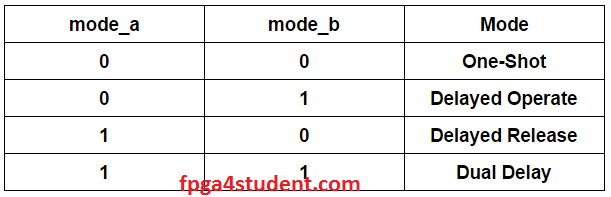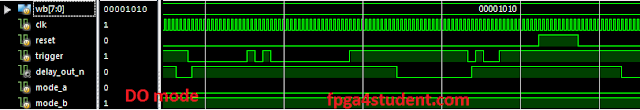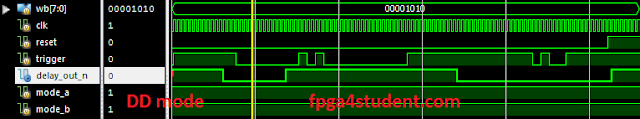# Delay timer (LS7212) in Verilog HDL

## Today's project is an implementation of a programmable digital delay timer in Verilog HDL. Verilog code for the delay timer is fully presented.#### The digital delay timer being implemented is CMOS IC LS7212 which is to generate programmable delays. The specification of the delay timer can be easily found here. Basically, the delay timer has 4 operating modes: one-shot (OS), Delayed Operate (DO), Delayed Release(DR), Dual Delay (DD). Those four modes will be selected by inputs mode_a and mode_b.The wb[7:0] input is to program the delays according to given equations in the specification of the delay timer. To understand more how it works in each mode and how inputs control delays, kindly refer to its datasheet. The following waveform is how the delays are generated in each mode.### Below is the Verilog code for the programmable digital delay timer LS7212.

``` //fpga4student.com: FPga projects, Verilog projects, VHDL projects
// Verilog project: Verilog code for delay timer LS7212
module delay_timer_ls7212
(
input [7:0] wb, // weighting bits
input clk, // clock input
input reset, // timer reset
input trigger, // trigger input
input mode_a, mode_b, // mode bits A and B
output reg delay_out_n // delay output, active low
);
reg[7:0] PULSE_WIDTH ;
reg [7:0] DELAY;
reg [7:0] TIMER=0;
reg trigger_sync_1=0,trigger_sync_2=0;
wire trigger_rising,trigger_falling;
reg timer_start=0,out_low=0;
wire timer_clear2,timer_clear3,timer_clear;
reg [1:0] mode;
reg reset_timer1=0,reset_timer2=0,reset_timer=0;
wire reset_timer3,reset_det;
reg reset_det1=0,reset_det2=0;
//fpga4student.com: FPga projects, Verilog projects, VHDL projects
always @(posedge clk)
begin
trigger_sync_1 <= trigger; // the first Flip-Flop
trigger_sync_2 <= trigger_sync_1;// the second Flip-Flop
reset_timer1 <= reset_timer;
reset_timer2 <= reset_timer1;
reset_det1 <= reset;
reset_det2 <= reset_det1;
end
// Identify the zero to one transitions on trigger signal
assign trigger_rising = trigger_sync_1 & (~trigger_sync_2);
assign trigger_falling = trigger_sync_2 & (~trigger_sync_1);
assign reset_timer3 = reset_timer1 & (~reset_timer2);
assign reset_det = reset_det2 & (~reset_det1);
// sample Mode and wb
always @(trigger_rising,trigger_falling,mode_a,mode_b,wb)
begin
if(trigger_falling == 1 || trigger_rising == 1) begin
PULSE_WIDTH = wb;
DELAY = (2*wb + 1)/2;
mode = {mode_a,mode_b};
end
end
// modes
always @(mode,reset,trigger_falling,trigger_rising,TIMER,reset,trigger,PULSE_WIDTH,DELAY,reset_det)
begin
case(mode)
2'b00: // One-Shot Mode
begin
if(reset) begin
out_low <= 0;
timer_start <= 0;
reset_timer <= 1;
end
else if(trigger_rising==1) begin
out_low <= 1;
timer_start <= 1;
reset_timer <= 1;
end
else if(TIMER>=PULSE_WIDTH) begin
out_low <= 0;
timer_start <= 0;
reset_timer <= 1;
end
end
2'b01: // Delayed Operate Mode
begin
if(reset) begin
out_low <= 0;
timer_start <= 0;
reset_timer <= 1;
end
else if(reset_det==1 && trigger==1) begin
timer_start <= 1;
reset_timer <= 0;
end
else if(trigger_rising==1) begin
timer_start <= 1;
reset_timer <= 0;
end
else if(trigger_falling==1 || trigger == 0) begin
out_low <= 0;
reset_timer <= 1;
timer_start <= 0;
end
else if(TIMER >= DELAY) begin
out_low <= 1;
timer_start <= 0;
reset_timer <= 1;
end
//else
//     reset_timer <= 0;
end
2'b10: // Delayed Release Mode
begin
if(reset) begin
out_low <= 0;
timer_start <= 0;
reset_timer <= 1;
end
else if(trigger_rising==1 || trigger == 1) begin
out_low <= 1;
end
else if(trigger_falling==1 ) begin
timer_start <= 1;
reset_timer <= 0;
end
else if(TIMER>=DELAY) begin
out_low <= 0;
timer_start <= 0;
reset_timer <= 1;
end
end
2'b11: // Delayed Dual Mode
begin
if(reset) begin
out_low <= 0;
timer_start <= 0;
reset_timer <= 1;
end
else if(reset_det==1 && trigger==1) begin
timer_start <= 1;
reset_timer <= 0;
end
else if(trigger_falling==1 || trigger_rising==1 ) begin
timer_start <= 1;
reset_timer <= 0;
end
else if(TIMER>=DELAY) begin
out_low <= trigger;
timer_start <= 0;
reset_timer <= 1;
end
end
endcase
end
//fpga4student.com: FPga projects, Verilog projects, VHDL projects
// timer
always @(posedge clk or posedge timer_clear)
begin
if(timer_clear)
TIMER <= 0;
else if(timer_start)
TIMER <= TIMER + 1;
end
assign timer_clear = reset_timer3 | trigger_rising == 1 | timer_clear3 ;
assign timer_clear2 = (trigger_rising == 1)|(trigger_falling == 1);
assign timer_clear3 = timer_clear2 & (mode == 2'b11);
//delay output
always @(posedge clk)
begin
if(out_low == 1)
delay_out_n <= 0;
else
delay_out_n <= 1;
end
endmodule
```

### Testbench Verilog code for the delay timer:

````timescale 1ns / 1ps
//fpga4student.com: FPga projects, Verilog projects, VHDL projects
// Testbench Verilog code for delay timer
module tb_ls7212;
// Inputs
reg [7:0] wb;
reg clk;
reg reset;
reg trigger;
reg mode_a;
reg mode_b;
// Outputs
wire delay_out_n;
//fpga4student.com: FPga projects, Verilog projects, VHDL projects
// Instantiate the Unit Under Test (UUT)
delay_timer_ls7212 uut (
.wb(wb),
.clk(clk),
.reset(reset),
.trigger(trigger),
.mode_a(mode_a),
.mode_b(mode_b),
.delay_out_n(delay_out_n)
);
initial begin
// Initialize Inputs
wb = 10;
mode_a = 0;
mode_b = 0;
reset = 0;
trigger = 0;
#500;
trigger = 1;
#15000;
trigger = 0;
#15000;
trigger = 1;
#2000;
trigger = 0;
#2000;
trigger = 1;
#2000;
trigger = 0;
#20000;
trigger = 1;
#30000;
trigger = 0;
#2000;
trigger = 1;
#2000;
trigger = 0;
#4000;
trigger = 1;
#10000;
reset = 1;
#10000;
reset = 0;
// Delay Operate
end
initial begin
clk = 0;
forever #500 clk = ~clk;
end
endmodule
//fpga4student.com: FPga projects, Verilog projects, VHDL projects
```

#### One-Shot Mode#### Delayed Operate Mode#### Delayed Release Mode#### Delay Dual ModeThe Verilog code for the programmable delay timer is synthesizable and can be implemented on FPGAs.

#### 1 comment:

1.There seem to a 1 cycle delay in delay_out_n response.